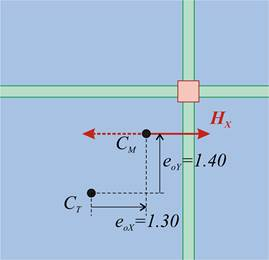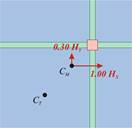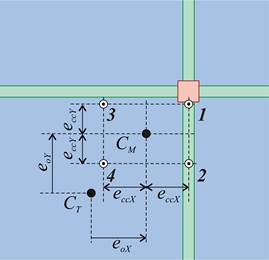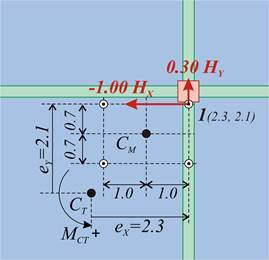The structural frame
The construction
The reinforcement I
The reinforcement II
Quantity/Cost estimation
Detailing drawings
Introduction >Wind and Seismic Forces >
Structural model and Analysis
Slabs
Seismic behavour of frames
Appendix A
Appendix B
Appendix C
Appendix D
Introduction >
Modelling slabsMaterials
To be continued >
IntroductionFigure 6.5-1: Typical floor plan. CM is the centre of mass CT is the centre of stiffness Figure 6.5-2: Detail of the CM, CT region eoX, eoY are the structural eccentricities

The main horizontal seismic force HX is applied at each diaphragm of a building due to the seismic action along the axis X of the building. This force is applied at the diaphragm’s centre of mass CM and generates the moment MCTΧ of magnitude |HX×eoY| about the centre of stiffness CT. The same situation exists  for the Y axis where the corresponding moment MCTY has a magnitude equal to |HY×eoX|. The sign of seismic forces changes continuously resulting in combinations having either positive or negative values of them. The magnitude of bending moments depends however on the size of the structural eccentricities eoX, eoY. The position of the centre of stiffness and thus of the structural eccentricities is examined in §5.4 and in the Appendices C and D.

## Loading combination table for a specific location of the centre of massFigure 6.5-3 B: +1.00EX + 0.30EY Figure 6.5-4 C: +1.00EX - 0.30EY Figure 6.5-5 D: +0.30EX + 1.00EY Figure 6.5-6 E: -0.30EX + 1.00EYFigure 6.5-7 F: -1.00EX - 0.30EY Figure 6.5-8 G: -1.00EX + 0.30EY Figure 6.5-9 H: -0.30EX – 1.00EY Figure 6.5-10 I: +0.30EX – 1.00EY

Regardless of the CM, CT locations the combination A of gravity loads g and q multiplied by the safety factors of actions always exists . For any specific location of CM, CT, 8 additional combinations of the seismic forces exist, due to the most probable masses 1.00g+0.30q during the earthquake.

## Table of the most adverse relative locations of centres of mass and stiffnessFigure 6.5-11: The possible locations of CΤ as to CΜ (or equivalent of CM as to CT) Figure 6.5-12: Example of the adverse combination 1G MCT=-1.00HX×eY+0.30HY×eX